# Rajasthan Board Question Papers for Class 12th Maths 2017 With Solutions In PDF

Rajasthan Class 12 Maths 2017 question paper with solutions are provided here in a downloadable pdf format and also in the text, so that the students can obtain an accurate explanation for all the questions. Along with the solutions, maths question paper 2017 Class 12 is also available here for reference. Students are able to access all the Rajasthan board previous year maths question papers. Students are advised to first solve these RBSE Class 12 Maths Previous Year Question Paper 2017 and then to refer to the solutions, so that they can analyse their exam preparations.

The solutions provided by BYJU’S will assist the students to get an idea on the kind of questions asked in the board exams. The solutions give a clear picture as to how to present their answers in the exams. Solving the question papers will guide the students in a better way by helping them to evaluate their preparation level.

### QUESTION PAPER CODE 816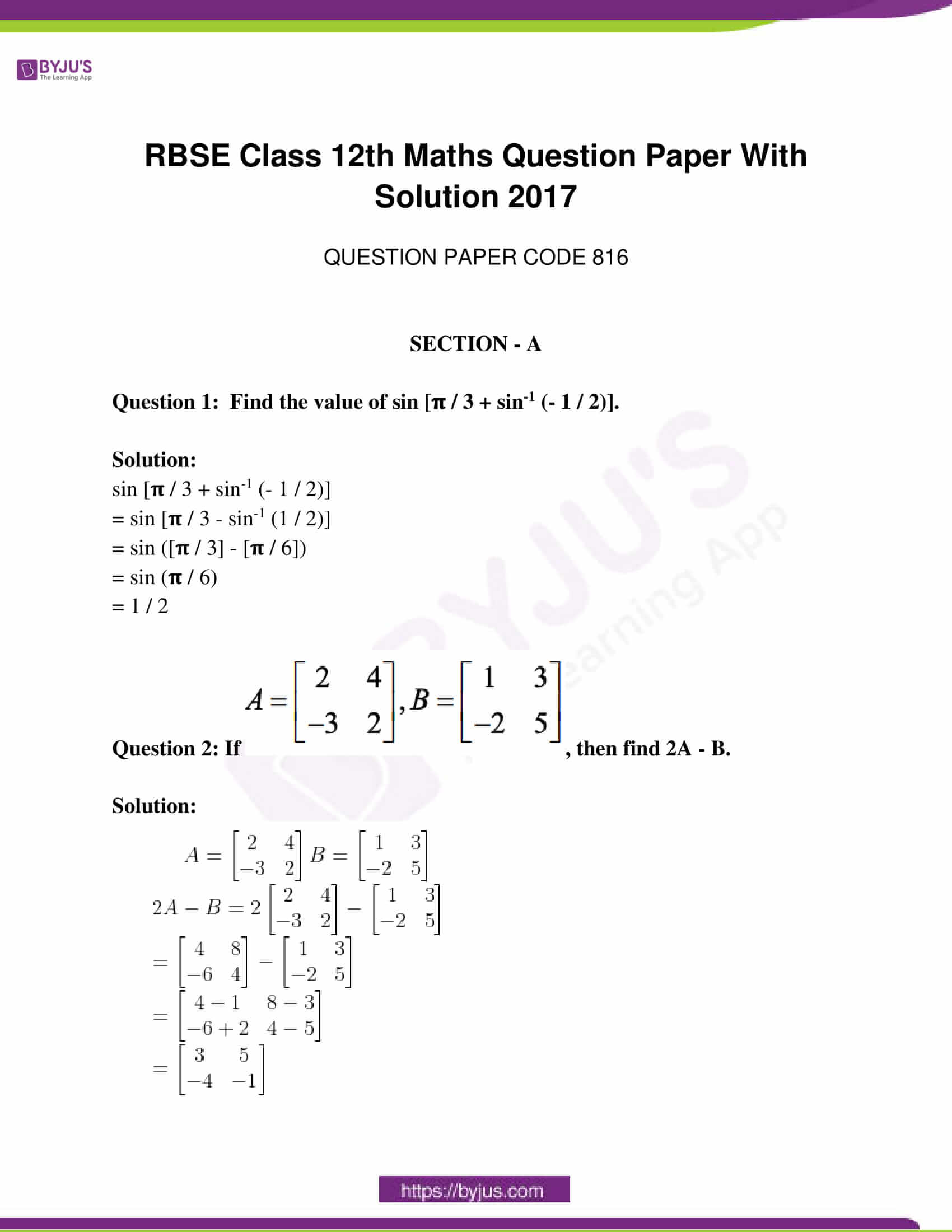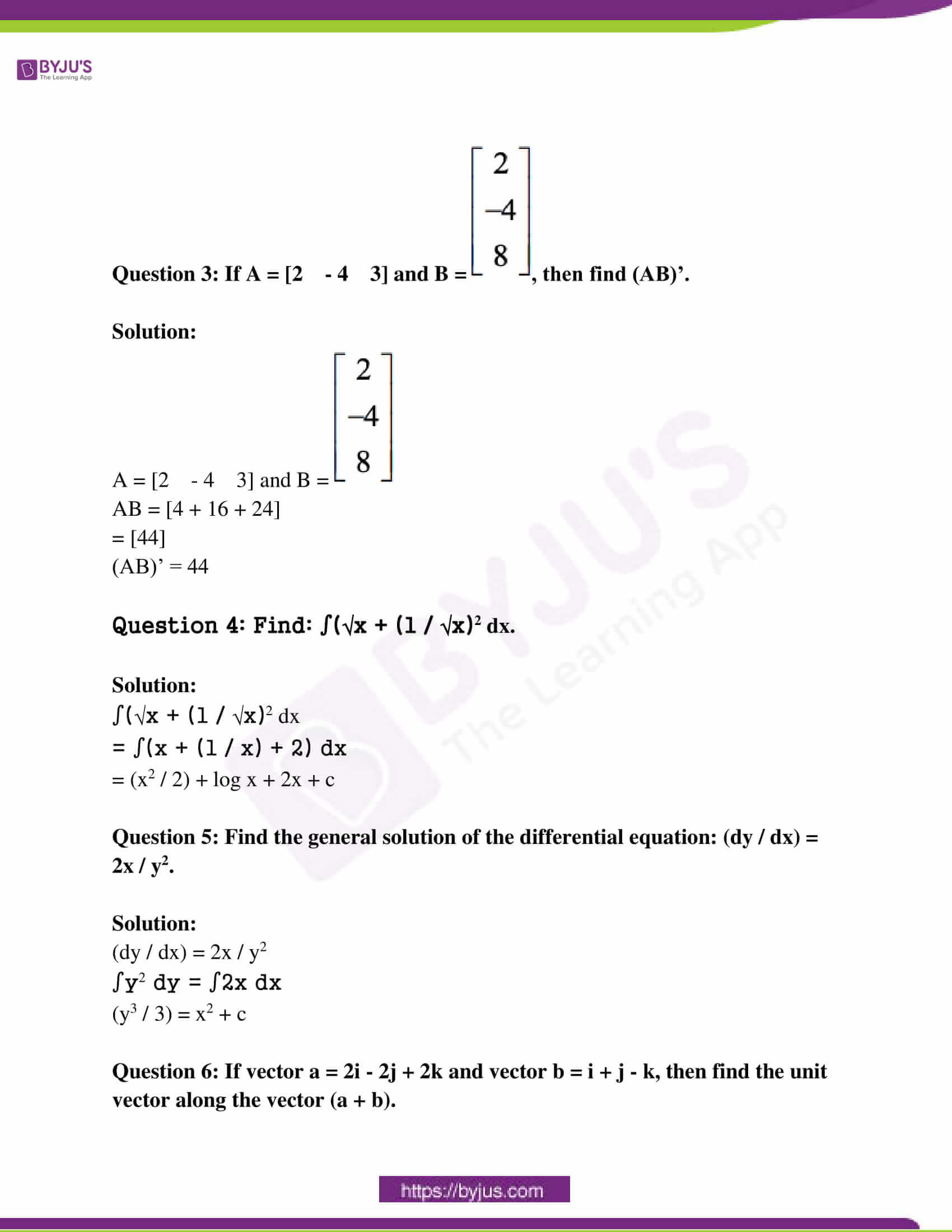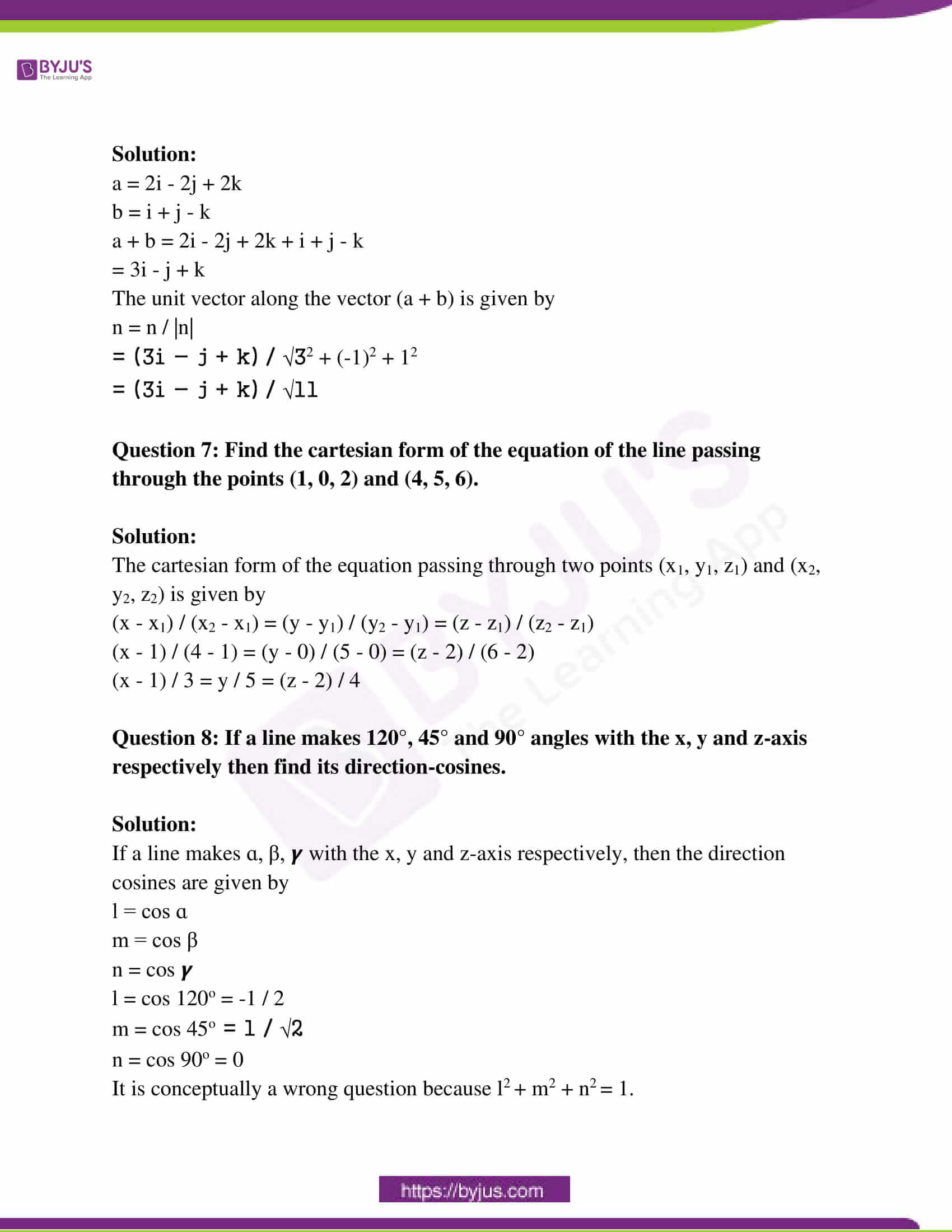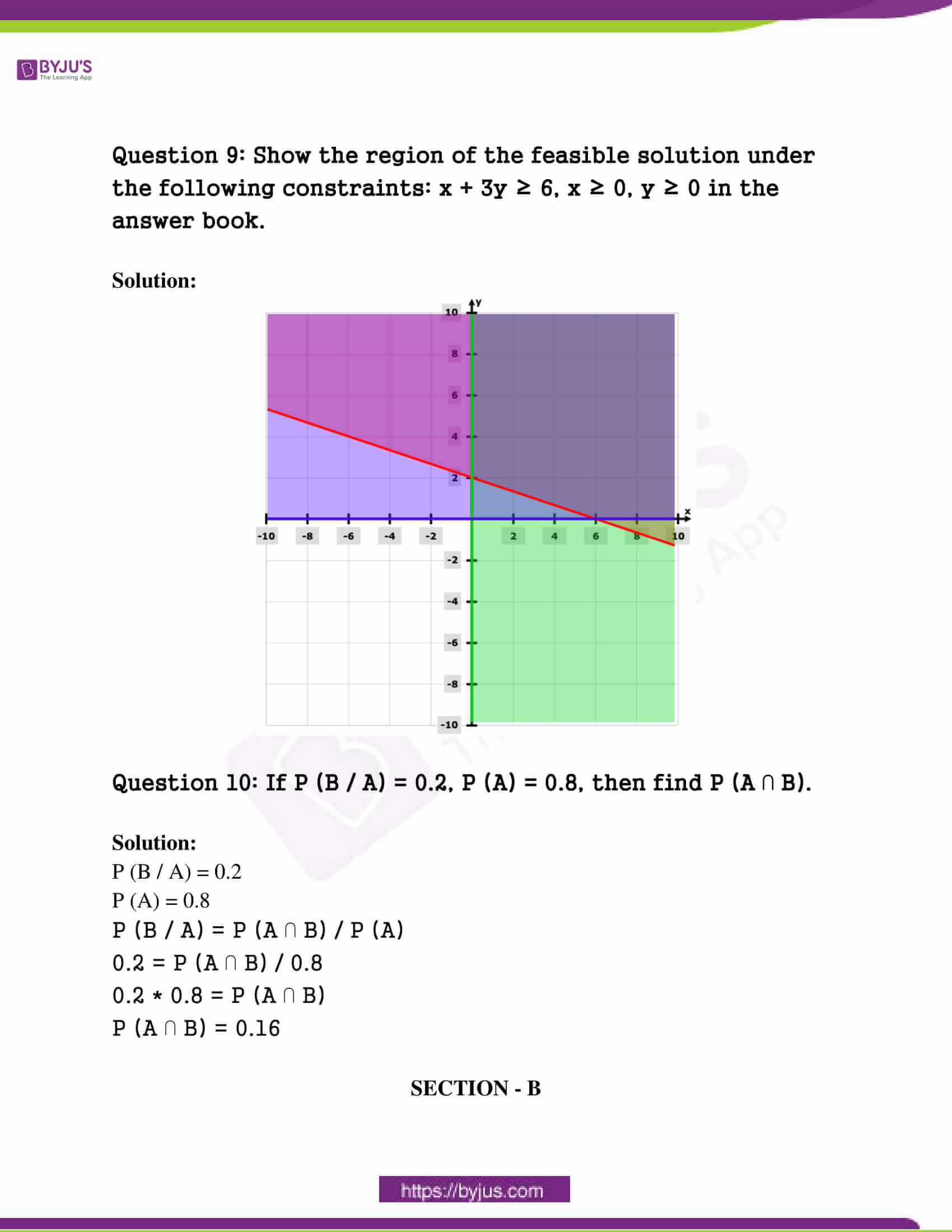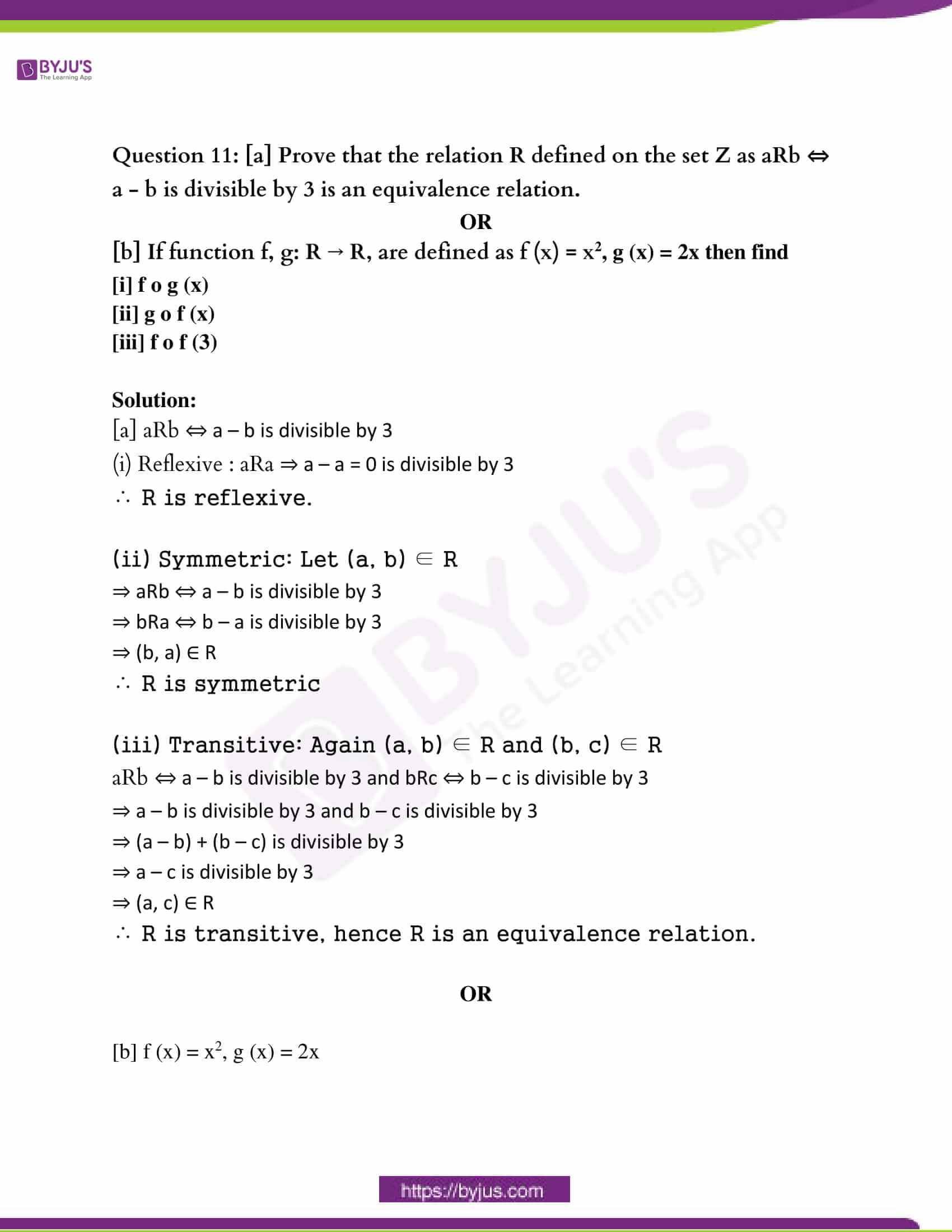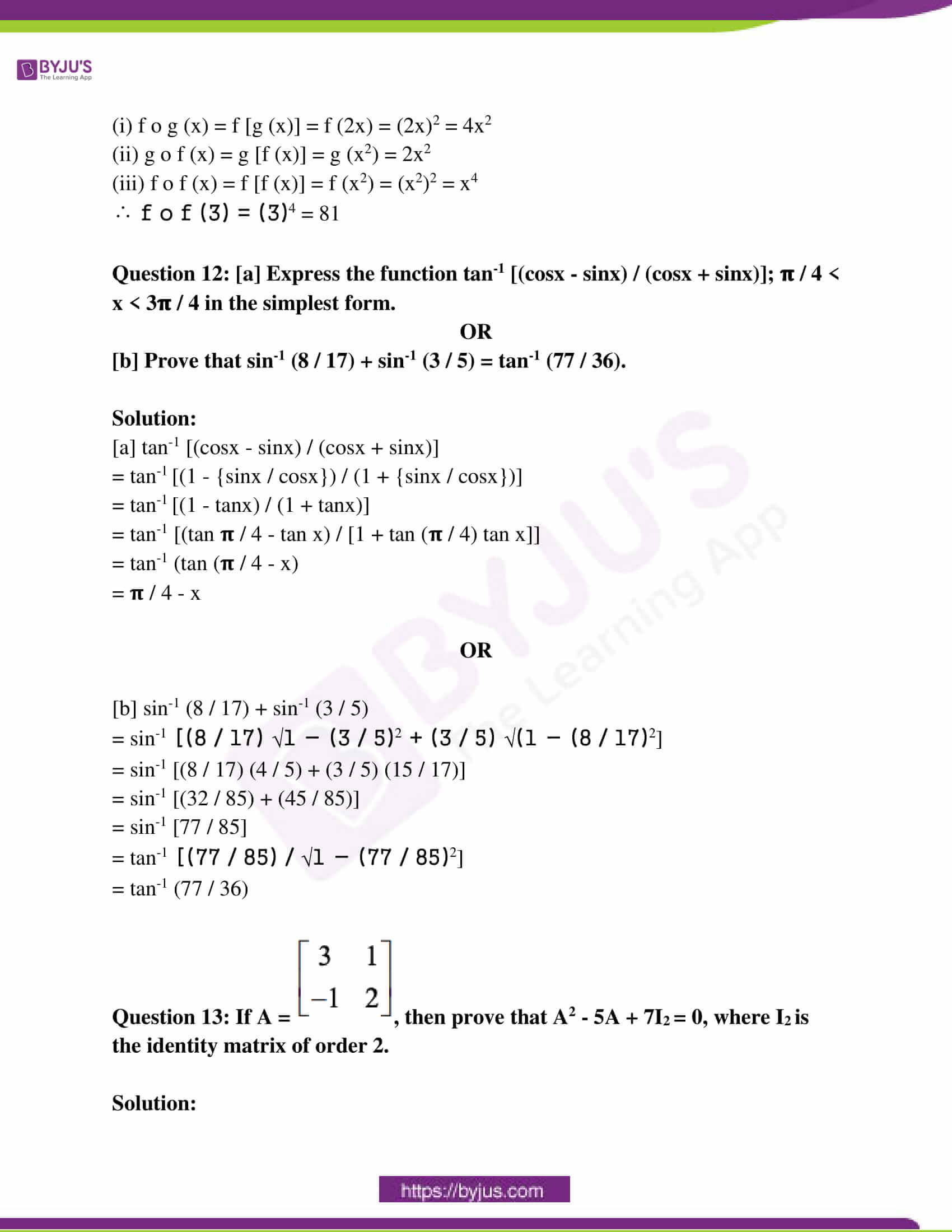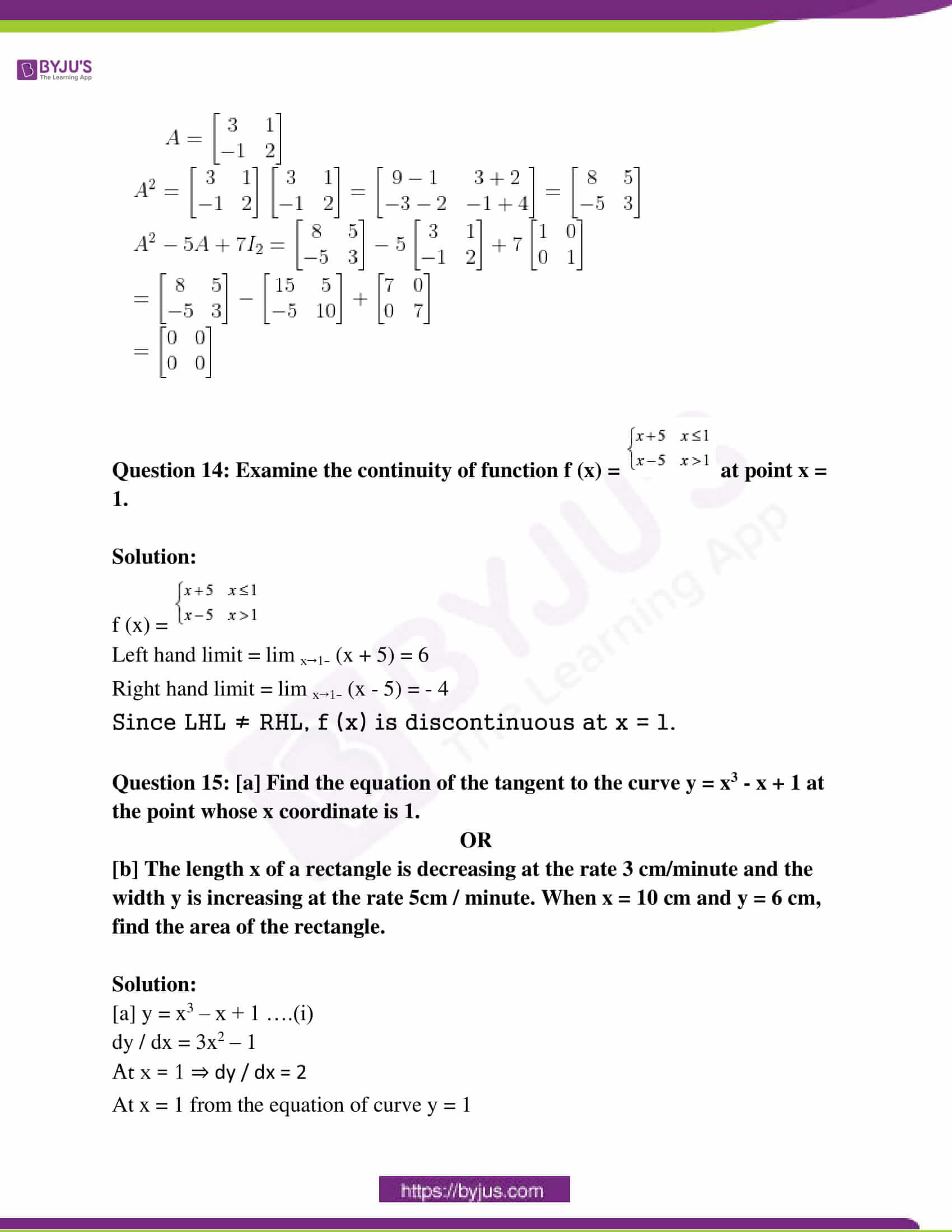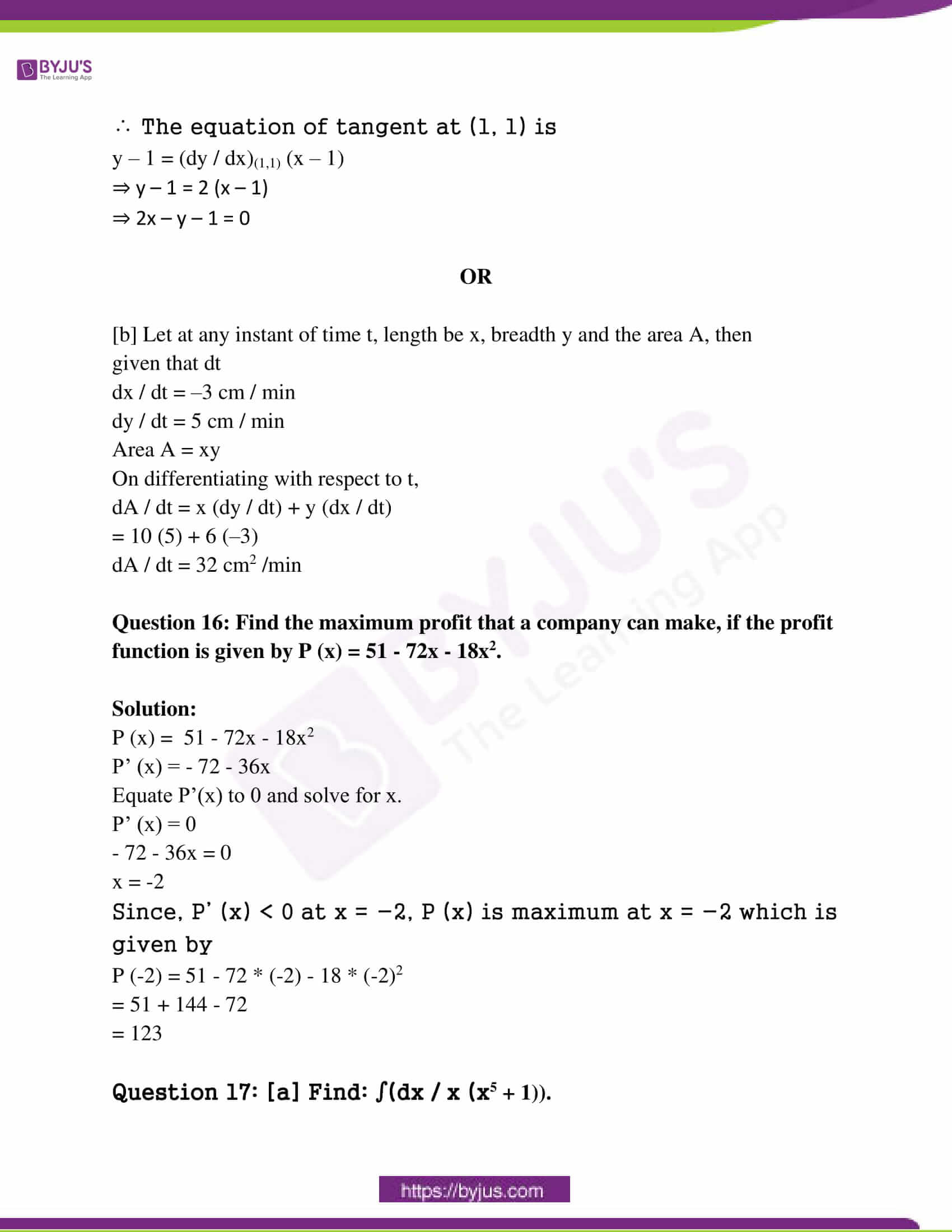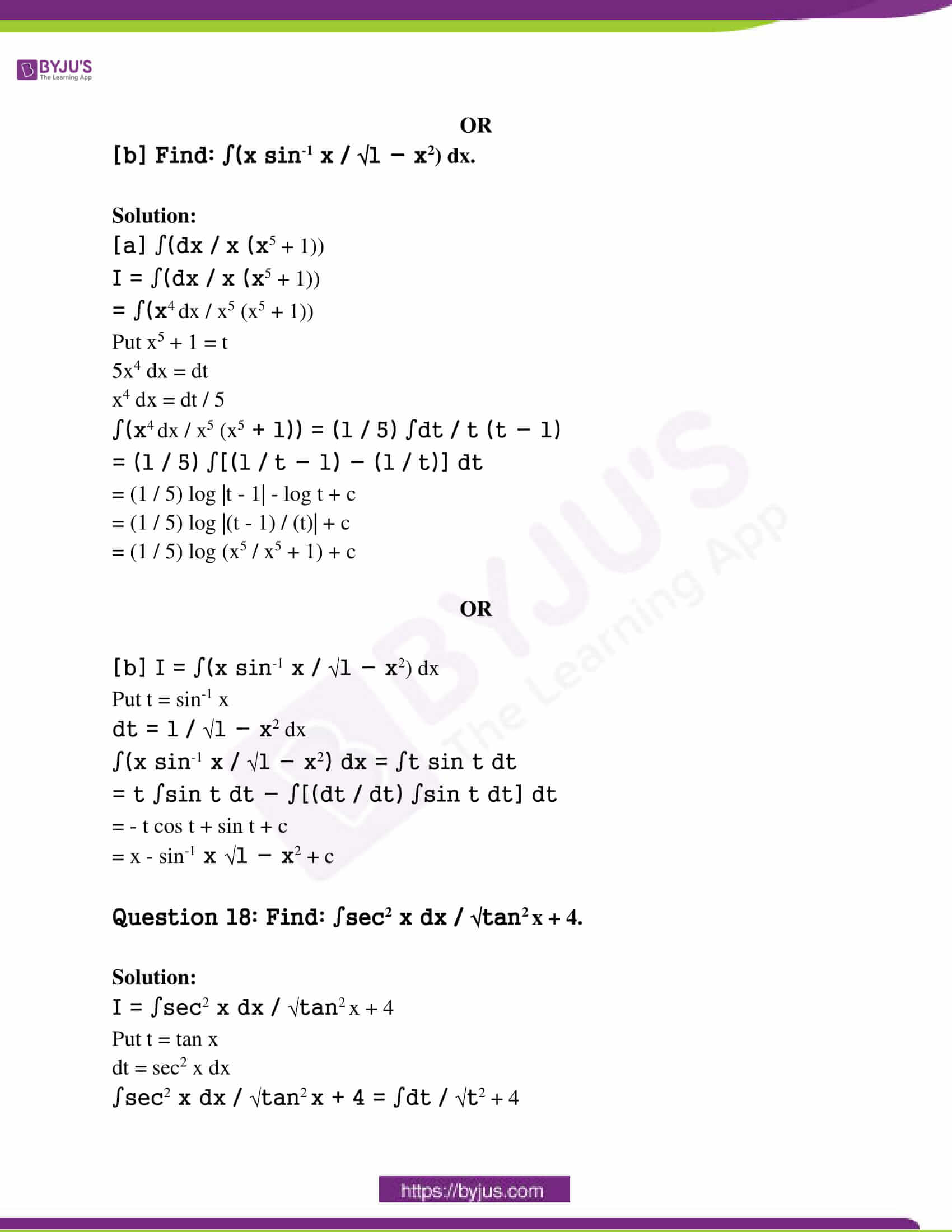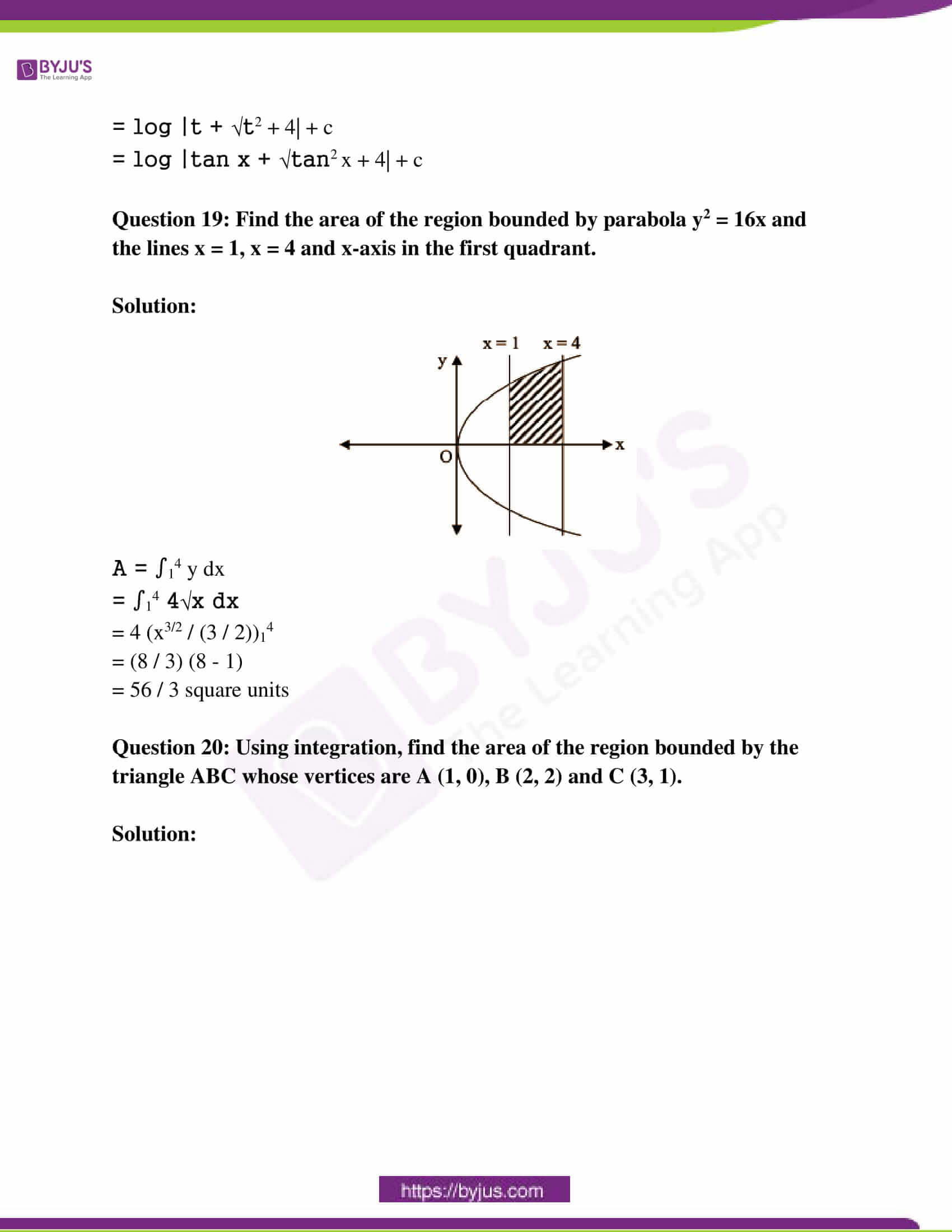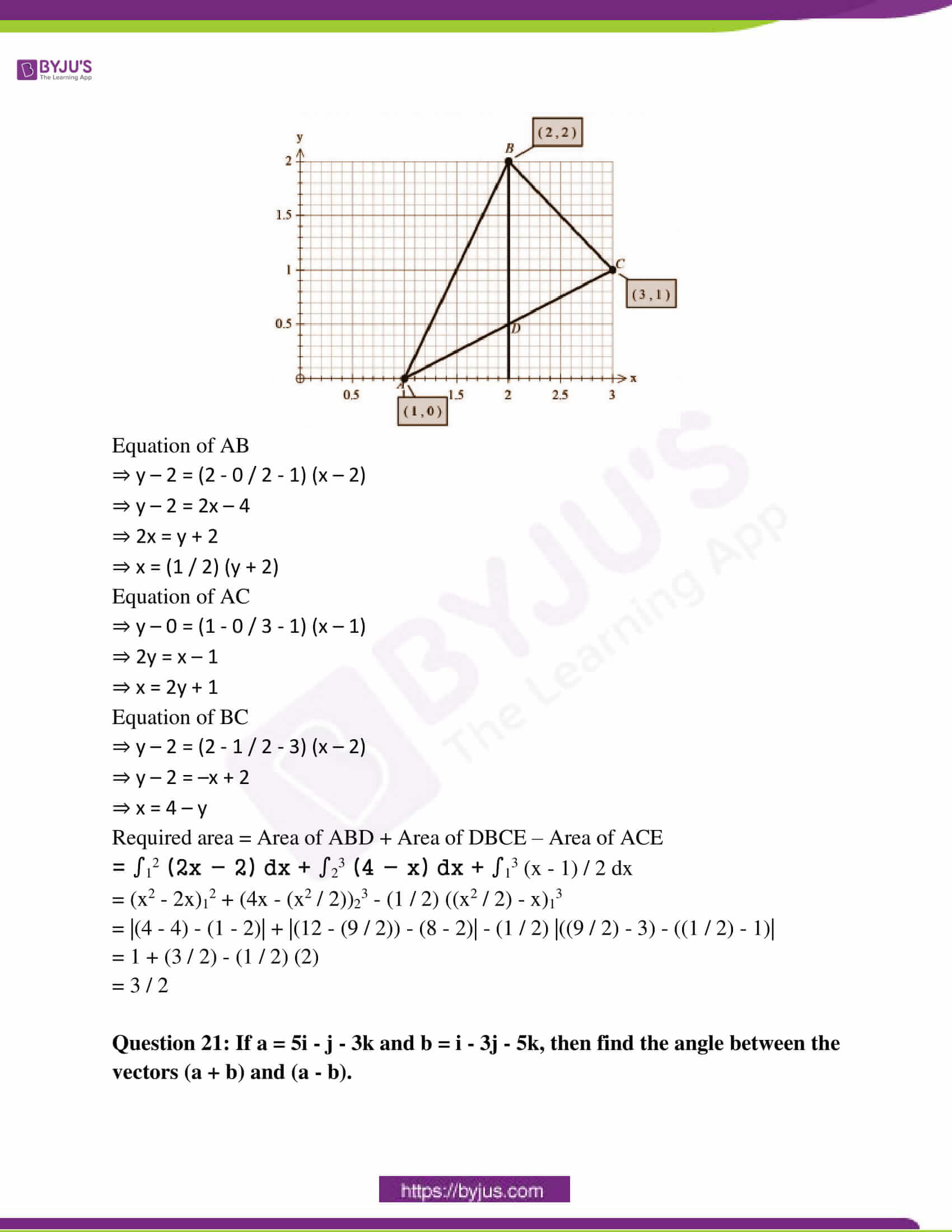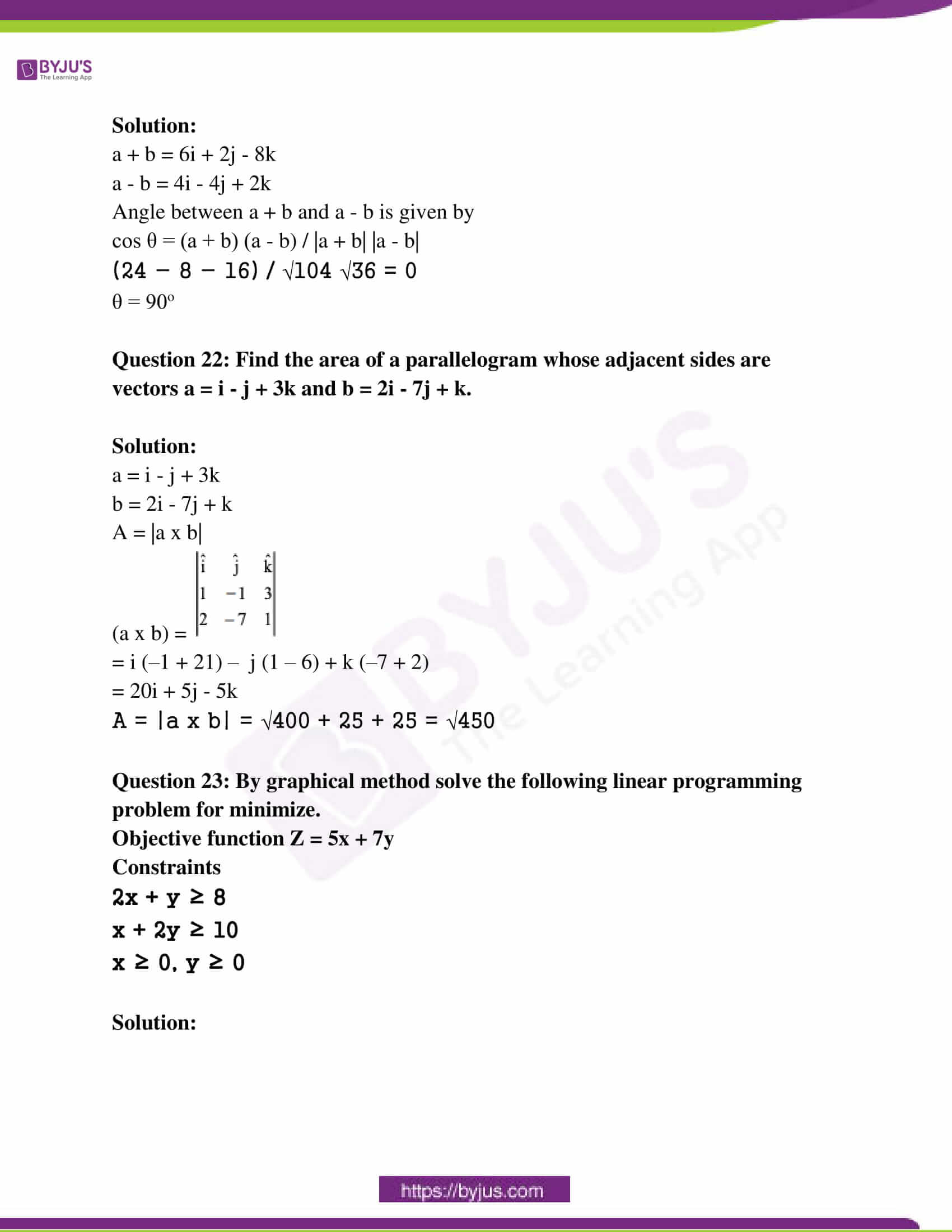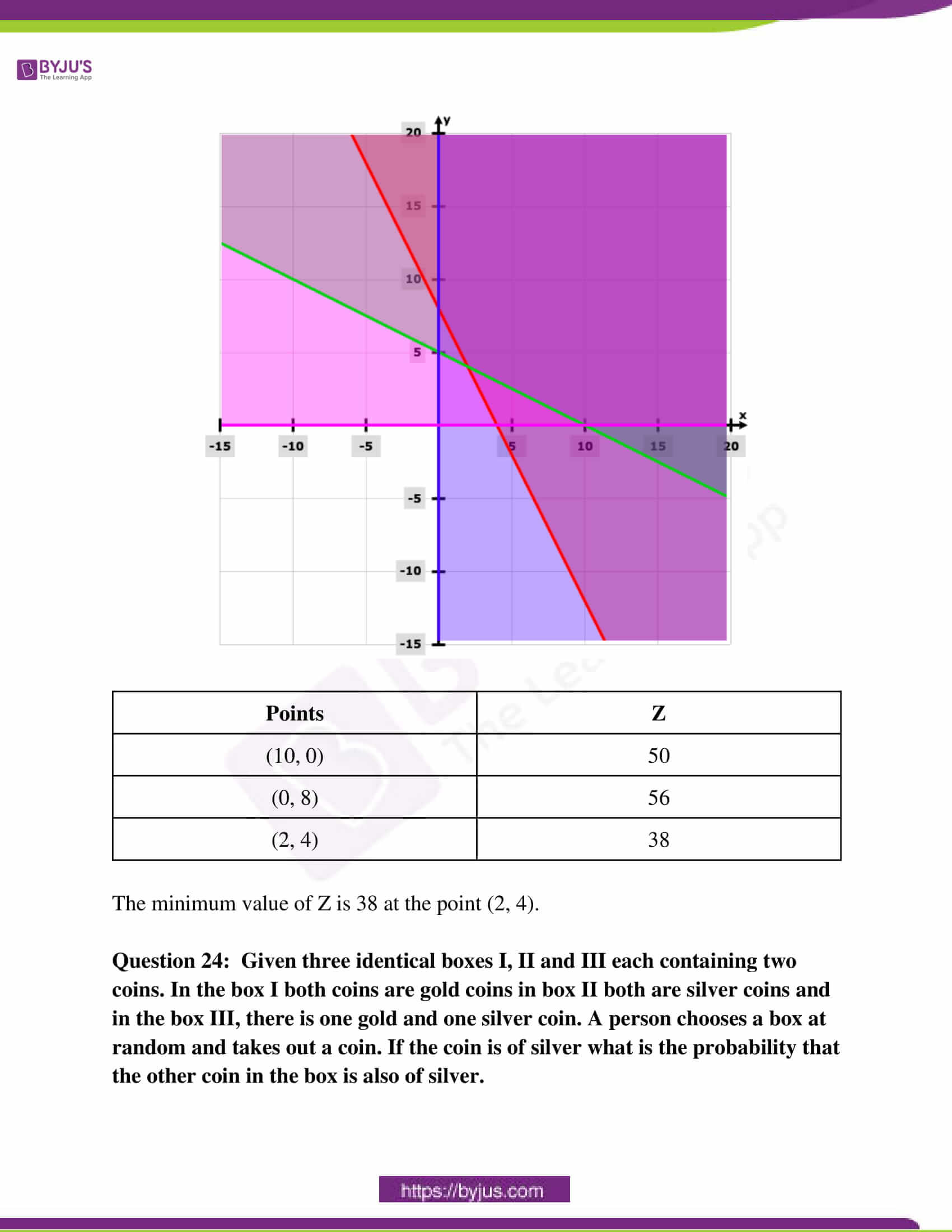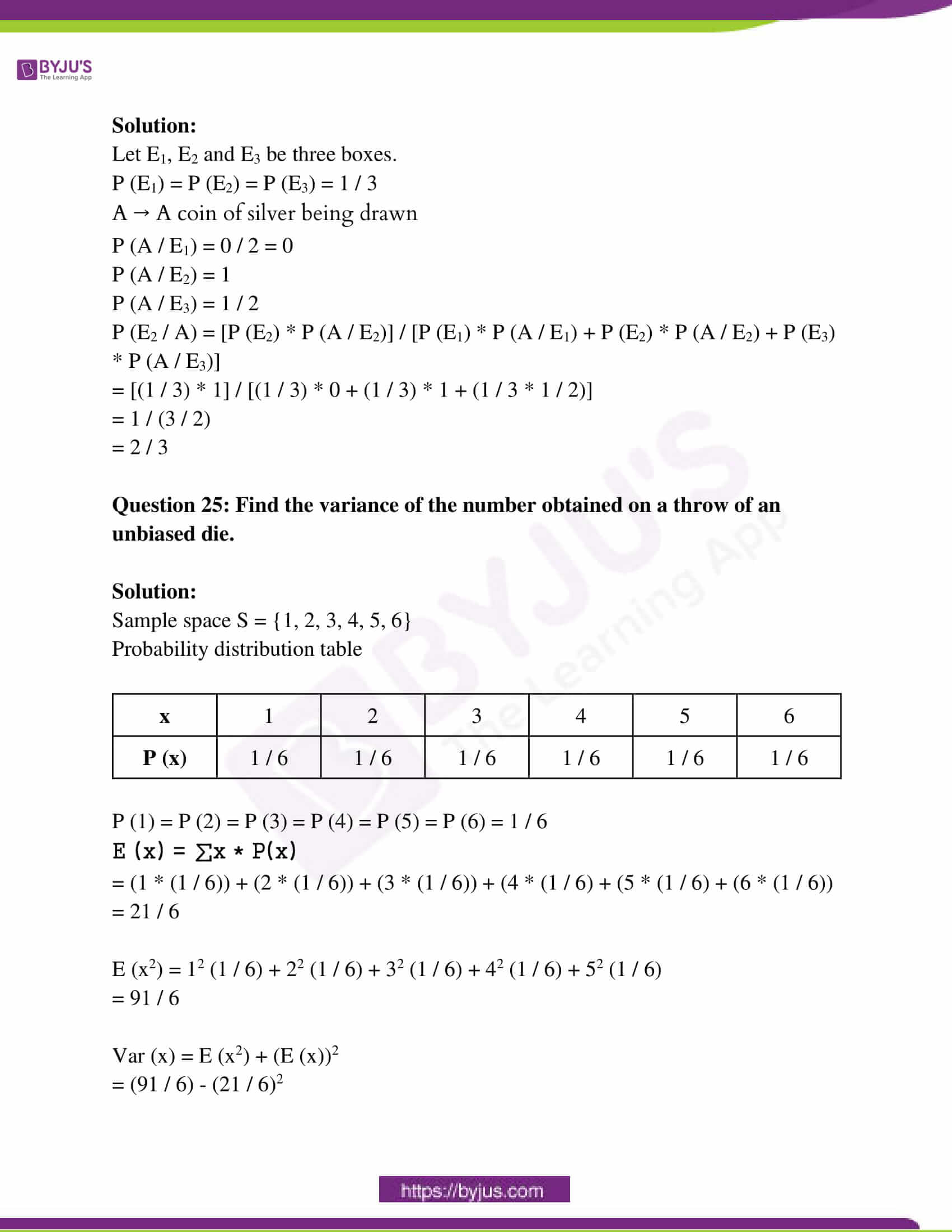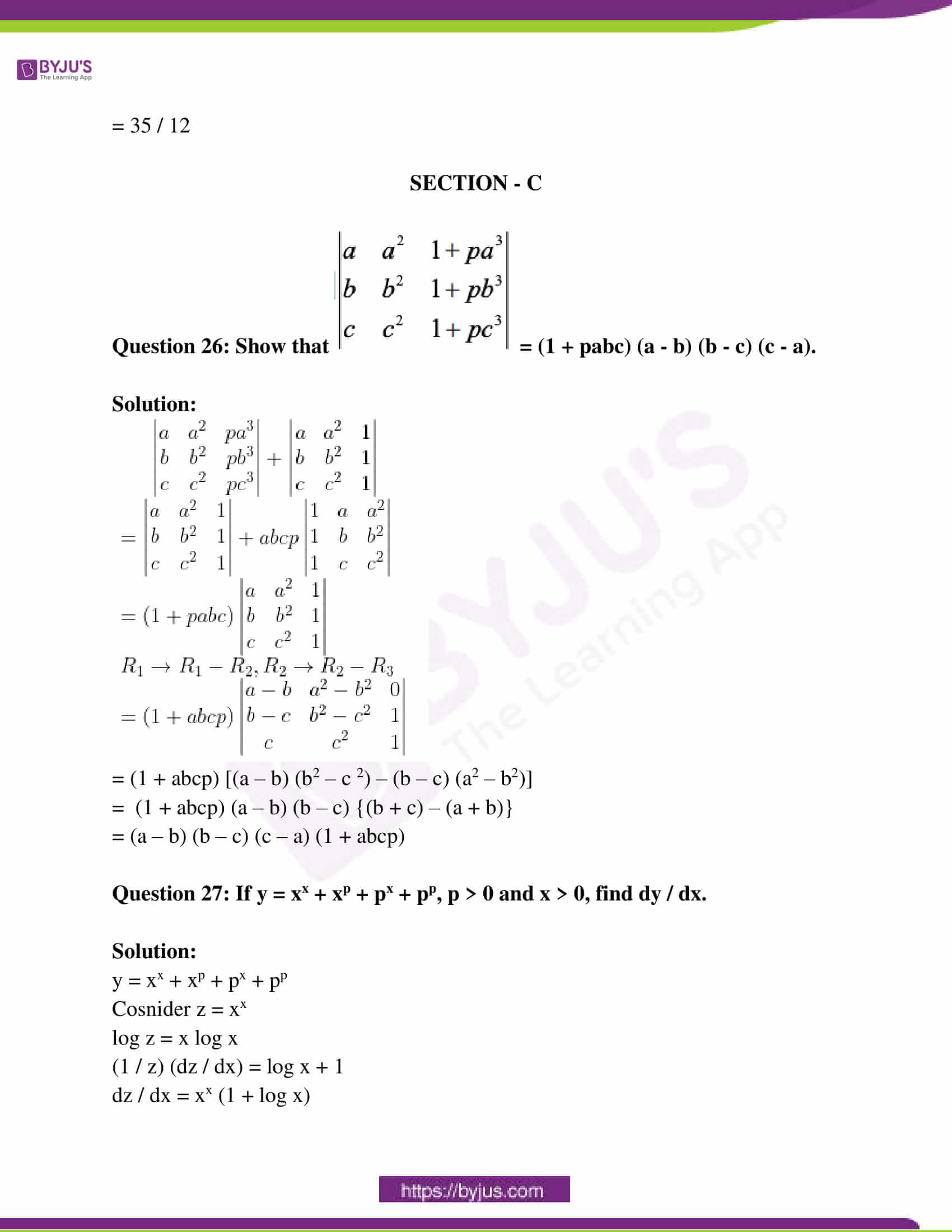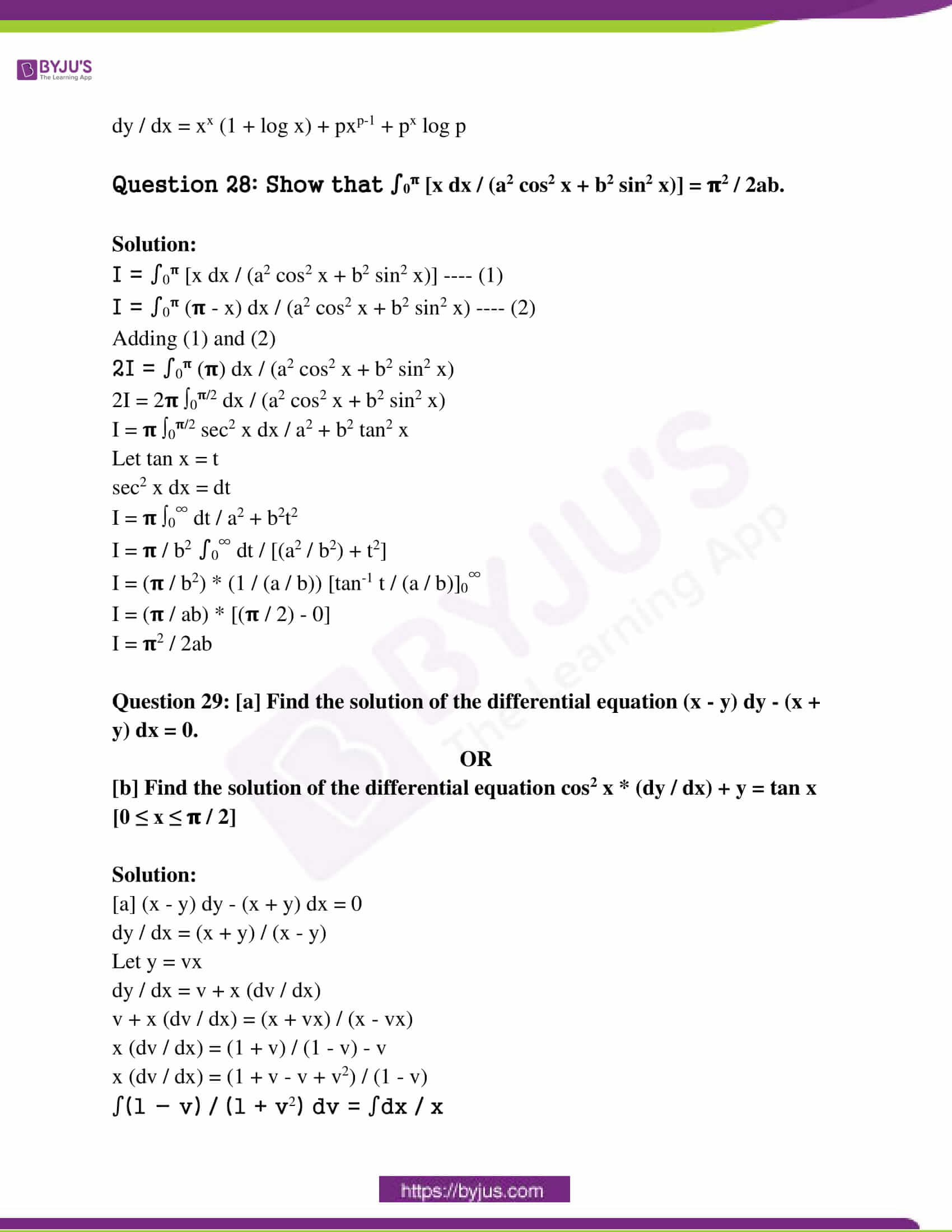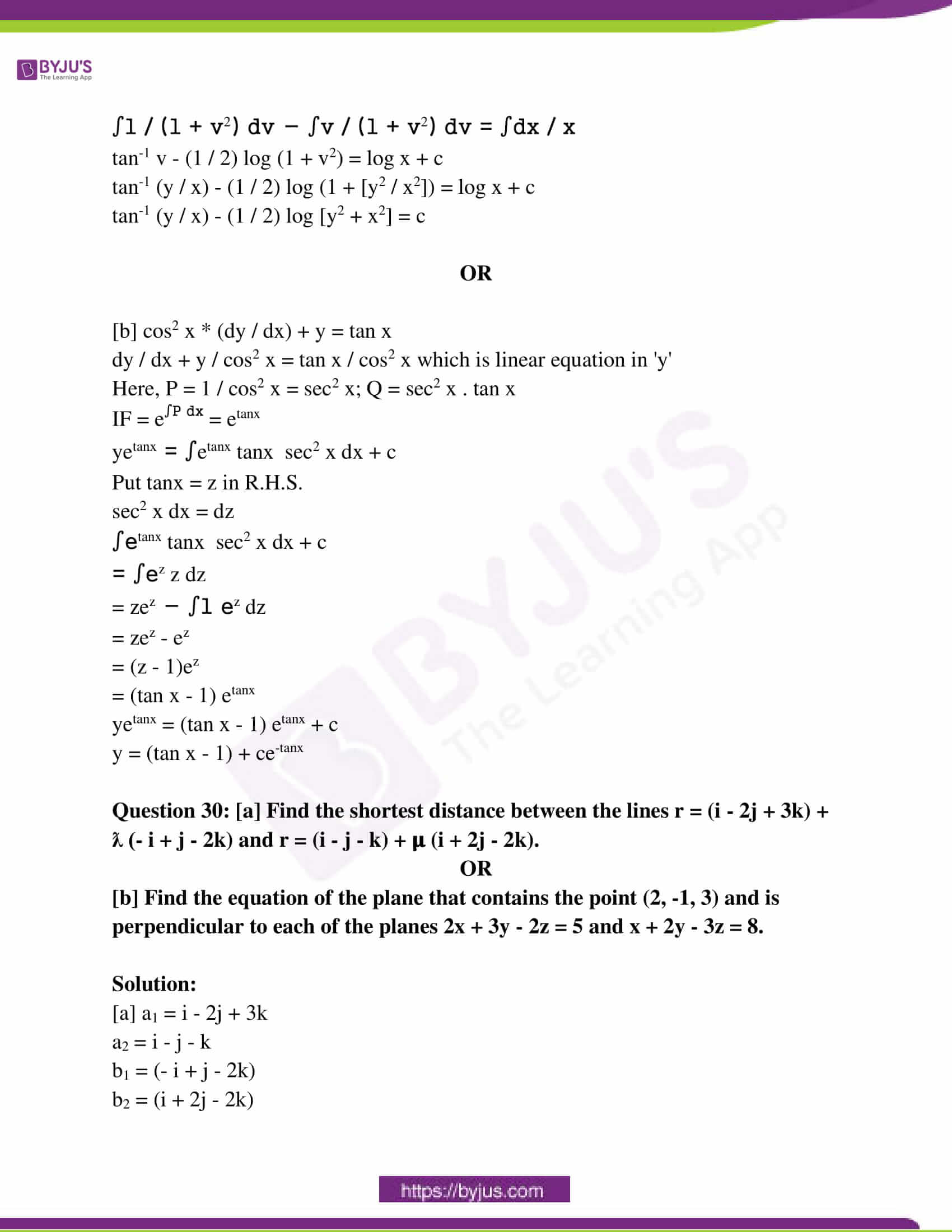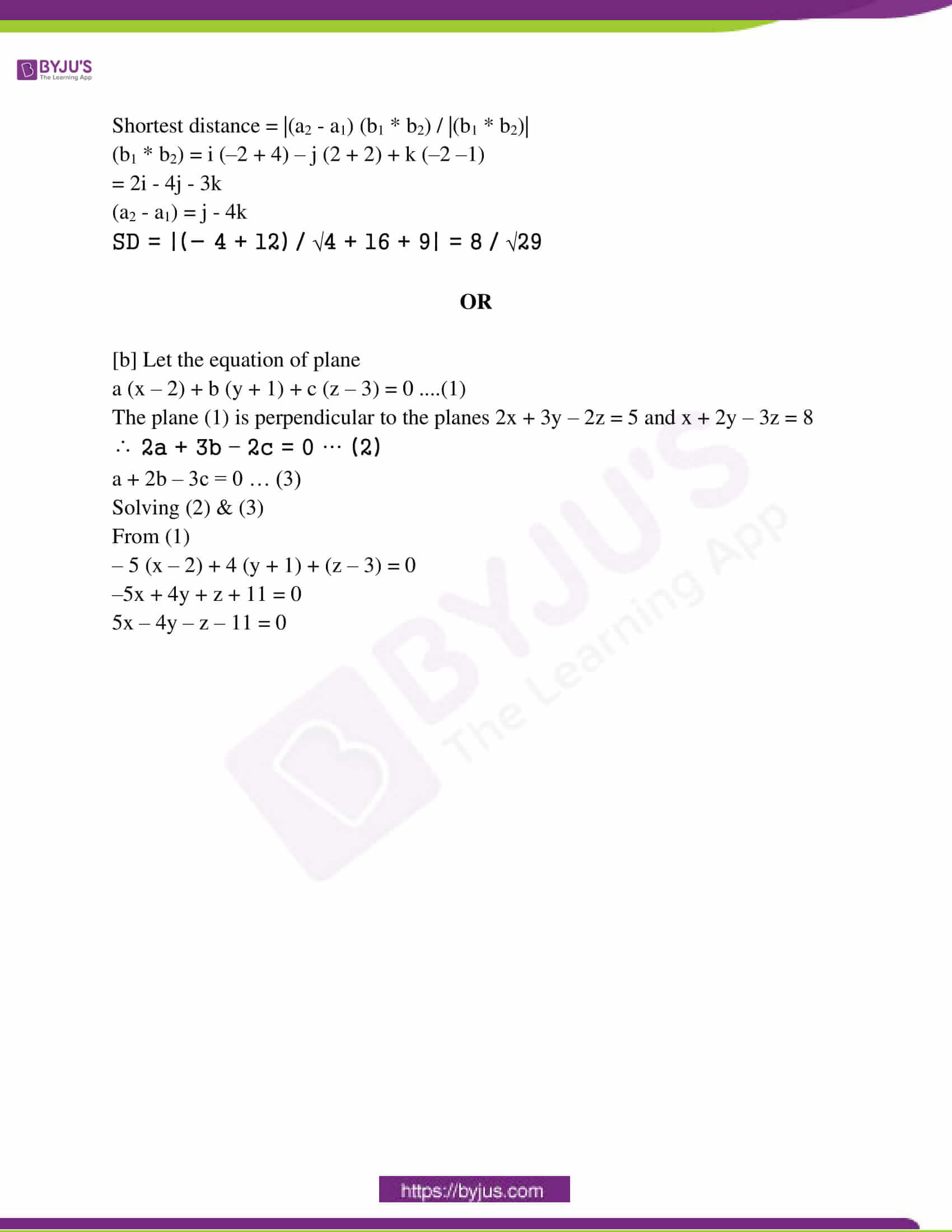SECTION – A

Question 1: Find the value of sin [𝛑 / 3 + sin-1 (- 1 / 2)].

Solution:

sin [𝛑 / 3 + sin-1 (- 1 / 2)]

= sin [𝛑 / 3 – sin-1 (1 / 2)]

= sin ([𝛑 / 3] – [𝛑 / 6])

= sin (𝛑 / 6)

= 1 / 2

Question 2: If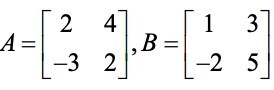, then find 2A – B.

Solution: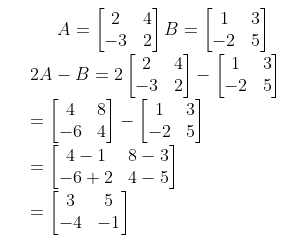Question 3: If A = [2 – 4 3] and B =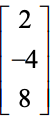, then find (AB)’.

Solution:

A = [2 – 4 3] and B =AB = [4 + 16 + 24]

= 

(AB)’ = 44

Question 4: Find: ∫(√x + (1 / √x)2 dx.

Solution:

∫(√x + (1 / √x)2 dx

= ∫(x + (1 / x) + 2) dx

= (x2 / 2) + log x + 2x + c

Question 5: Find the general solution of the differential equation: (dy / dx) = 2x / y2.

Solution:

(dy / dx) = 2x / y2

∫y2 dy = ∫2x dx

(y3 / 3) = x2 + c

Question 6: If vector a = 2i – 2j + 2k and vector b = i + j – k, then find the unit vector along the vector (a + b).

Solution:

a = 2i – 2j + 2k

b = i + j – k

a + b = 2i – 2j + 2k + i + j – k

= 3i – j + k

The unit vector along the vector (a + b) is given by

n = n / |n|

= (3i – j + k) / √32 + (-1)2 + 12

= (3i – j + k) / √11

Question 7: Find the cartesian form of the equation of the line passing through the points (1, 0, 2) and (4, 5, 6).

Solution:

The cartesian form of the equation passing through two points (x1, y1, z1) and (x2, y2, z2) is given by

(x – x1) / (x2 – x1) = (y – y1) / (y2 – y1) = (z – z1) / (z2 – z1)

(x – 1) / (4 – 1) = (y – 0) / (5 – 0) = (z – 2) / (6 – 2)

(x – 1) / 3 = y / 5 = (z – 2) / 4

Question 8: If a line makes 120°, 45° and 90° angles with the x, y and z-axis, respectively then find its direction-cosines.

Solution:

If a line makes ɑ, β, 𝞬 with the x, y and z-axis respectively, then the direction cosines are given by

l = cos ɑ

m = cos β

n = cos 𝞬

l = cos 120o = -1 / 2

m = cos 45o = 1 / √2

n = cos 90o = 0

It is conceptually a wrong question because l2 + m2 + n2 = 1.

Question 9: Show the region of the feasible solution under the following constraints: x + 3y ≥ 6, x ≥ 0, y ≥ 0 in the answer book.

Solution: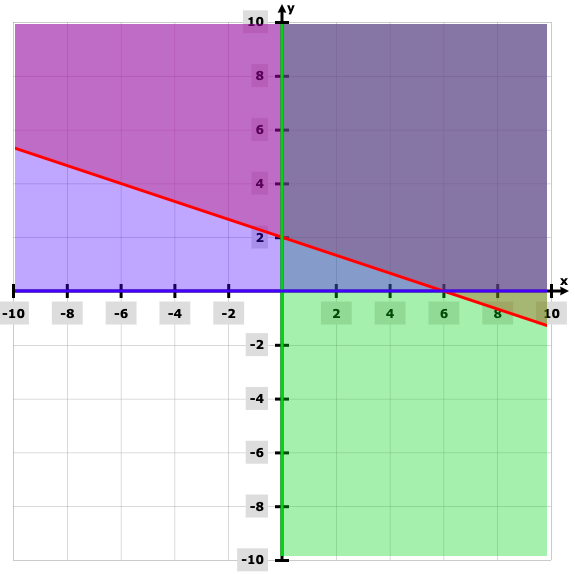Question 10: If P (B / A) = 0.2, P (A) = 0.8, then find P (A ⋂ B).

Solution:

P (B / A) = 0.2

P (A) = 0.8

P (B / A) = P (A ⋂ B) / P (A)

0.2 = P (A ⋂ B) / 0.8

0.2 * 0.8 = P (A ⋂ B)

P (A ⋂ B) = 0.16

SECTION – B

Question 11: [a] Prove that the relation R defined on the set Z as aRb ⇔ a – b is divisible by 3 is an equivalence relation.

OR

[b] If function f, g: R → R, are defined as f (x) = x2, g (x) = 2x then find

[i] f o g (x)

[ii] g o f (x)

[iii] f o f (3)

Solution:

[a] aRb ⇔ a – b is divisible by 3

(i) Reflexive : aRa ⇒ a – a = 0 is divisible by 3

∴ R is reflexive.

(ii) Symmetric: Let (a, b) ∈ R

⇒ aRb ⇔ a – b is divisible by 3

⇒ bRa ⇔ b – a is divisible by 3

⇒ (b, a) ∈ R

∴ R is symmetric

(iii) Transitive: Again (a, b) ∈ R and (b, c) ∈ R

aRb ⇔ a – b is divisible by 3 and bRc ⇔ b – c is divisible by 3

⇒ a – b is divisible by 3 and b – c is divisible by 3

⇒ (a – b) + (b – c) is divisible by 3

⇒ a – c is divisible by 3

⇒ (a, c) ∈ R

∴ R is transitive, hence R is an equivalence relation.

OR

[b] f (x) = x2, g (x) = 2x

(i) f o g (x) = f [g (x)] = f (2x) = (2x)2 = 4x2

(ii) g o f (x) = g [f (x)] = g (x2) = 2x2

(iii) f o f (x) = f [f (x)] = f (x2) = (x2)2 = x4

∴ f o f (3) = (3)4 = 81

Question 12: [a] Express the function tan-1 [(cosx – sinx) / (cosx + sinx)]; 𝛑 / 4 < x < 3𝛑 / 4 in the simplest form.

OR

[b] Prove that sin-1 (8 / 17) + sin-1 (3 / 5) = tan-1 (77 / 36).

Solution:

[a] tan-1 [(cosx – sinx) / (cosx + sinx)]

= tan-1 [(1 – {sinx / cosx}) / (1 + {sinx / cosx})]

= tan-1 [(1 – tanx) / (1 + tanx)]

= tan-1 [(tan 𝛑 / 4 – tan x) / [1 + tan (𝛑 / 4) tan x]]

= tan-1 (tan (𝛑 / 4 – x)

= 𝛑 / 4 – x

OR

[b] sin-1 (8 / 17) + sin-1 (3 / 5)

= sin-1 [(8 / 17) √1 – (3 / 5)2 + (3 / 5) √(1 – (8 / 17)2]

= sin-1 [(8 / 17) (4 / 5) + (3 / 5) (15 / 17)]

= sin-1 [(32 / 85) + (45 / 85)]

= sin-1 [77 / 85]

= tan-1 [(77 / 85) / √1 – (77 / 85)2]

= tan-1 (77 / 36)

Question 13: If A =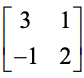, then prove that A2 – 5A + 7I2 = 0, where I2 is the identity matrix of order 2.

Solution: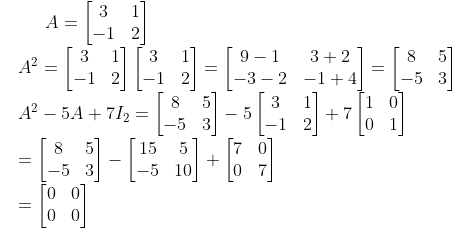Question 14: Examine the continuity of function f (x) =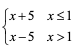at point x = 1.

Solution:

f (x) =Left hand limit = lim x→1- (x + 5) = 6

Right hand limit = lim x→1- (x – 5) = – 4

Since LHL ≠ RHL, f (x) is discontinuous at x = 1.

Question 15: [a] Find the equation of the tangent to the curve y = x3 – x + 1 at the point whose x coordinate is 1.

OR

[b] The length x of a rectangle is decreasing at the rate 3 cm/minute and the width y is increasing at the rate 5cm / minute. When x = 10 cm and y = 6 cm, find the area of the rectangle.

Solution:

[a] y = x3 – x + 1 ….(i)

dy / dx = 3x2 – 1

At x = 1 ⇒ dy / dx = 2

At x = 1 from the equation of curve y = 1

∴ The equation of tangent at (1, 1) is

y – 1 = (dy / dx)(1,1) (x – 1)

⇒ y – 1 = 2 (x – 1)

⇒ 2x – y – 1 = 0

OR

[b] Let at any instant of time t, length be x, breadth y and the area A, then

given that dt

dx / dt = –3 cm / min

dy / dt = 5 cm / min

Area A = xy

On differentiating with respect to t,

dA / dt = x (dy / dt) + y (dx / dt)

= 10 (5) + 6 (–3)

dA / dt = 32 cm2 /min

Question 16: Find the maximum profit that a company can make, if the profit function is given by P (x) = 51 – 72x – 18x2.

Solution:

P (x) = 51 – 72x – 18x2

P’ (x) = – 72 – 36x

Equate P’(x) to 0 and solve for x.

P’ (x) = 0

– 72 – 36x = 0

x = -2

Since, P’ (x) < 0 at x = -2, P (x) is maximum at x = −2 which is given by

P (-2) = 51 – 72 * (-2) – 18 * (-2)2

= 51 + 144 – 72

= 123

Question 17: [a] Find: ∫(dx / x (x5 + 1)).

OR

[b] Find: ∫(x sin-1 x / √1 – x2) dx.

Solution:

[a] ∫(dx / x (x5 + 1))

I = ∫(dx / x (x5 + 1))

= ∫(x4 dx / x5 (x5 + 1))

Put x5 + 1 = t

5x4 dx = dt

x4 dx = dt / 5

∫(x4 dx / x5 (x5 + 1)) = (1 / 5) ∫dt / t (t – 1)

= (1 / 5) ∫[(1 / t – 1) – (1 / t)] dt

= (1 / 5) log |t – 1| – log t + c

= (1 / 5) log |(t – 1) / (t)| + c

= (1 / 5) log (x5 / x5 + 1) + c

OR

[b] I = ∫(x sin-1 x / √1 – x2) dx

Put t = sin-1 x

dt = 1 / √1 – x2 dx

∫(x sin-1 x / √1 – x2) dx = ∫t sin t dt

= t ∫sin t dt – ∫[(dt / dt) ∫sin t dt] dt

= – t cos t + sin t + c

= x – sin-1 x √1 – x2 + c

Question 18: Find: ∫sec2 x dx / √tan2 x + 4.

Solution:

I = ∫sec2 x dx / √tan2 x + 4

Put t = tan x

dt = sec2 x dx

∫sec2 x dx / √tan2 x + 4 = ∫dt / √t2 + 4

= log |t + √t2 + 4| + c

= log |tan x + √tan2 x + 4| + c

Question 19: Find the area of the region bounded by parabola y2 = 16x and the lines x = 1, x = 4 and x-axis in the first quadrant.

Solution: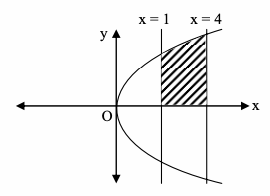A = ∫14 y dx

= ∫14 4√x dx

= 4 (x3/2 / (3 / 2))14

= (8 / 3) (8 – 1)

= 56 / 3 square units

Question 20: Using integration, find the area of the region bounded by the triangle ABC whose vertices are A (1, 0), B (2, 2) and C (3, 1).

Solution: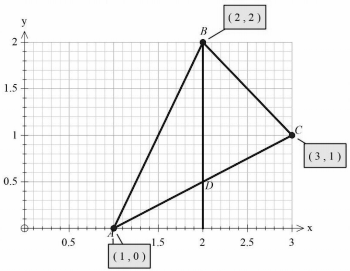Equation of AB

⇒ y – 2 = (2 – 0 / 2 – 1) (x – 2)

⇒ y – 2 = 2x – 4

⇒ 2x = y + 2

⇒ x = (1 / 2) (y + 2)

Equation of AC

⇒ y – 0 = (1 – 0 / 3 – 1) (x – 1)

⇒ 2y = x – 1

⇒ x = 2y + 1

Equation of BC

⇒ y – 2 = (2 – 1 / 2 – 3) (x – 2)

⇒ y – 2 = –x + 2

⇒ x = 4 – y

Required area = Area of ABD + Area of DBCE – Area of ACE

= ∫12 (2x – 2) dx + ∫23 (4 – x) dx + ∫13 (x – 1) / 2 dx

= (x2 – 2x)12 + (4x – (x2 / 2))23 – (1 / 2) ((x2 / 2) – x)13

= |(4 – 4) – (1 – 2)| + |(12 – (9 / 2)) – (8 – 2)| – (1 / 2) |((9 / 2) – 3) – ((1 / 2) – 1)|

= 1 + (3 / 2) – (1 / 2) (2)

= 3 / 2

Question 21: If a = 5i – j – 3k and b = i – 3j – 5k, then find the angle between the vectors (a + b) and (a – b).

Solution:

a + b = 6i + 2j – 8k

a – b = 4i – 4j + 2k

Angle between a + b and a – b is given by

cos θ = (a + b) (a – b) / |a + b| |a – b|

(24 – 8 – 16) / √104 √36 = 0

θ = 90o

Question 22: Find the area of a parallelogram whose adjacent sides are vectors a = i – j + 3k and b = 2i – 7j + k.

Solution:

a = i – j + 3k

b = 2i – 7j + k

A = |a x b|

(a x b) =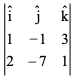= i (–1 + 21) – j (1 – 6) + k (–7 + 2)

= 20i + 5j – 5k

A = |a x b| = √400 + 25 + 25 = √450

Question 23: By graphical method solve the following linear programming problem for minimize.

Objective function Z = 5x + 7y

Constraints

2x + y ≥ 8

x + 2y ≥ 10

x ≥ 0, y ≥ 0

Solution: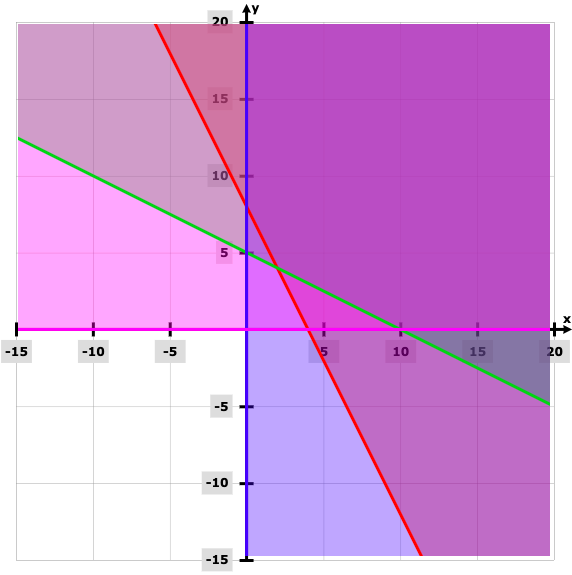Points Z (10, 0) 50 (0, 8) 56 (2, 4) 38

The minimum value of Z is 38 at the point (2, 4).

Question 24: Given three identical boxes I, II and III each containing two coins. In the box I both coins are gold coins in box II both are silver coins and in the box III, there is one gold and one silver coin. A person chooses a box at random and takes out a coin. If the coin is of silver what is the probability that the other coin in the box is also of silver.

Solution:

Let E1, E2 and E3 be three boxes.

P (E1) = P (E2) = P (E3) = 1 / 3

A → A coin of silver being drawn

P (A / E1) = 0 / 2 = 0

P (A / E2) = 1

P (A / E3) = 1 / 2

P (E2 / A) = [P (E2) * P (A / E2)] / [P (E1) * P (A / E1) + P (E2) * P (A / E2) + P (E3) * P (A / E3)]

= [(1 / 3) * 1] / [(1 / 3) * 0 + (1 / 3) * 1 + (1 / 3 * 1 / 2)]

= 1 / (3 / 2)

= 2 / 3

Question 25: Find the variance of the number obtained on a throw of an unbiased die.

Solution:

Sample space S = {1, 2, 3, 4, 5, 6}

Probability distribution table

 x 1 2 3 4 5 6 P (x) 1 / 6 1 / 6 1 / 6 1 / 6 1 / 6 1 / 6

P (1) = P (2) = P (3) = P (4) = P (5) = P (6) = 1 / 6

E (x) = ∑x * P(x)

= (1 * (1 / 6)) + (2 * (1 / 6)) + (3 * (1 / 6)) + (4 * (1 / 6) + (5 * (1 / 6) + (6 * (1 / 6))

= 21 / 6

E (x2) = 12 (1 / 6) + 22 (1 / 6) + 32 (1 / 6) + 42 (1 / 6) + 52 (1 / 6)

= 91 / 6

Var (x) = E (x2) + (E (x))2

= (91 / 6) – (21 / 6)2

= 35 / 12

SECTION – C

Question 26: Show that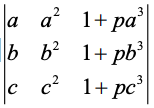= (1 + pabc) (a – b) (b – c) (c – a).

Solution: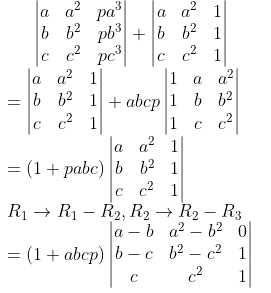= (1 + abcp) [(a – b) (b2 – c 2) – (b – c) (a2 – b2)]

= (1 + abcp) (a – b) (b – c) {(b + c) – (a + b)}

= (a – b) (b – c) (c – a) (1 + abcp)

Question 27: If y = xx + xp + px + pp, p > 0 and x > 0, find dy / dx.

Solution:

y = xx + xp + px + pp

Cosnider z = xx

log z = x log x

(1 / z) (dz / dx) = log x + 1

dz / dx = xx (1 + log x)

dy / dx = xx (1 + log x) + pxp-1 + px log p

Question 28: Show that ∫0𝛑 [x dx / (a2 cos2 x + b2 sin2 x)] = 𝛑2 / 2ab.

Solution:

I = ∫0𝛑 [x dx / (a2 cos2 x + b2 sin2 x)] —- (1)

I = ∫0𝛑 (𝛑 – x) dx / (a2 cos2 x + b2 sin2 x) —- (2)

2I = ∫0𝛑 (𝛑) dx / (a2 cos2 x + b2 sin2 x)

2I = 2𝛑 ∫0𝛑/2 dx / (a2 cos2 x + b2 sin2 x)

I = 𝛑 ∫0𝛑/2 sec2 x dx / a2 + b2 tan2 x

Let tan x = t

sec2 x dx = dt

I = 𝛑 ∫0 dt / a2 + b2t2

I = 𝛑 / b20 dt / [(a2 / b2) + t2]

I = (𝛑 / b2) * (1 / (a / b)) [tan-1 t / (a / b)]0

I = (𝛑 / ab) * [(𝛑 / 2) – 0]

I = 𝛑2 / 2ab

Question 29: [a] Find the solution of the differential equation (x – y) dy – (x + y) dx = 0.

OR

[b] Find the solution of the differential equation cos2 x * (dy / dx) + y = tan x [0 ≤ x ≤ 𝛑 / 2]

Solution:

[a] (x – y) dy – (x + y) dx = 0

dy / dx = (x + y) / (x – y)

Let y = vx

dy / dx = v + x (dv / dx)

v + x (dv / dx) = (x + vx) / (x – vx)

x (dv / dx) = (1 + v) / (1 – v) – v

x (dv / dx) = (1 + v – v + v2) / (1 – v)

∫(1 – v) / (1 + v2) dv = ∫dx / x

∫1 / (1 + v2) dv – ∫v / (1 + v2) dv = ∫dx / x

tan-1 v – (1 / 2) log (1 + v2) = log x + c

tan-1 (y / x) – (1 / 2) log (1 + [y2 / x2]) = log x + c

tan-1 (y / x) – (1 / 2) log [y2 + x2] = c

OR

[b] cos2 x * (dy / dx) + y = tan x

dy / dx + y / cos2 x = tan x / cos2 x which is linear equation in ‘y’

Here, P = 1 / cos2 x = sec2 x; Q = sec2 x . tan x

IF = e∫P dx = etanx

yetanx = ∫etanx tanx sec2 x dx + c

Put tanx = z in R.H.S.

sec2 x dx = dz

∫etanx tanx sec2 x dx + c

= ∫ez z dz

= zez – ∫1 ez dz

= zez – ez

= (z – 1)ez

= (tan x – 1) etanx

yetanx = (tan x – 1) etanx + c

y = (tan x – 1) + ce-tanx

Question 30: [a] Find the shortest distance between the lines r = (i – 2j + 3k) + ƛ (- i + j – 2k) and r = (i – j – k) + 𝛍 (i + 2j – 2k).

OR

[b] Find the equation of the plane that contains the point (2, -1, 3) and is perpendicular to each of the planes 2x + 3y – 2z = 5 and x + 2y – 3z = 8.

Solution:

[a] a1 = i – 2j + 3k

a2 = i – j – k

b1 = (- i + j – 2k)

b2 = (i + 2j – 2k)

Shortest distance = |(a2 – a1) (b1 * b2) / |(b1 * b2)|

(b1 * b2) = i (–2 + 4) – j (2 + 2) + k (–2 –1)

= 2i – 4j – 3k

(a2 – a1) = j – 4k

SD = |(- 4 + 12) / √4 + 16 + 9| = 8 / √29

OR

[b] Let the equation of plane

a (x – 2) + b (y + 1) + c (z – 3) = 0 ….(1)

The plane (1) is perpendicular to the planes 2x + 3y – 2z = 5 and x + 2y – 3z = 8

∴ 2a + 3b – 2c = 0 … (2)

a + 2b – 3c = 0 … (3)

Solving (2) & (3)

From (1)

– 5 (x – 2) + 4 (y + 1) + (z – 3) = 0

–5x + 4y + z + 11 = 0

5x – 4y – z – 11 = 0